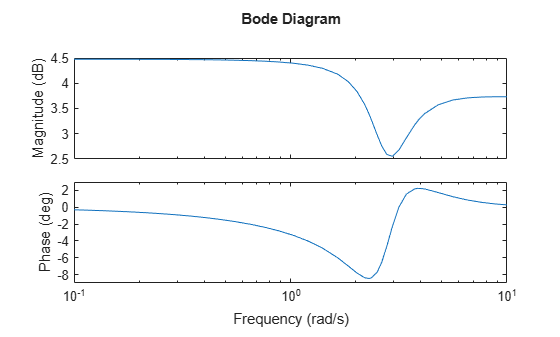Documentation

bodeplot

Plot Bode frequency response with additional plot customization options

Syntax

h = bodeplot(sys)
bodeplot(sys)
bodeplot(sys1,sys2,...)
bodeplot(AX,...)
bodeplot(..., plotoptions)
bodeplot(sys,w)

Description

h = bodeplot(sys) plot the Bode magnitude and phase of the dynamic system model sys and returns the plot handle h to the plot. You can use this handle to customize the plot with the getoptions and setoptions commands.

bodeplot(sys) draws the Bode plot of the model sys. The frequency range and number of points are chosen automatically.

bodeplot(sys1,sys2,...) graphs the Bode response of multiple models sys1,sys2,... on a single plot. You can specify a color, line style, and marker for each model, as in

bodeplot(sys1,'r',sys2,'y--',sys3,'gx')

bodeplot(AX,...) plots into the axes with handle AX.

bodeplot(..., plotoptions) plots the Bode response with the options specified in plotoptions. Type

help bodeoptions

for a list of available plot options. See Match Phase at Specified Frequency for an example of phase matching using the PhaseMatchingFreq and PhaseMatchingValue options.

bodeplot(sys,w) draws the Bode plot for frequencies specified by w. When w = {wmin,wmax}, the Bode plot is drawn for frequencies between wmin and wmax (in rad/TimeUnit, where TimeUnit is the time units of the input dynamic system, specified in the TimeUnit property of sys.). When w is a user-supplied vector w of frequencies, in rad/TimeUnit, the Bode response is drawn for the specified frequencies.

See logspace to generate logarithmically spaced frequency vectors.

Examples

collapse all

Generate a Bode plot.

h = bodeplot(sys);Change the units to Hz and suppress the phase plot. To do so, edit properties of the plot handle, h.

setoptions(h,'FreqUnits','Hz','PhaseVisible','off');The plot automatically updates when you call setoptions.

Create a Bode plot of a dynamic system.

sys = tf(1,[1 1]);
h = bodeplot(sys);Fix the phase at 1 rad/s to 750 degrees. To do so, get the plot properties. Then alter the properties PhaseMatchingFreq and PhaseMatchingValue to match a phase to a specified frequency.

p = getoptions(h);
p.PhaseMatching = 'on';
p.PhaseMatchingFreq = 1;
p.PhaseMatchingValue = 750;

Update the plot.

setoptions(h,p);The first bode plot has a phase of -45 degrees at a frequency of 1 rad/s. Setting the phase matching options so that at 1 rad/s the phase is near 750 degrees yields the second Bode plot. Note that, however, the phase can only be -45 + N*360, where N is an integer, and so the plot is set to the nearest allowable phase, namely 675 degrees (or 2*360 - 45 = 675).

Compare the frequency responses of identified state-space models of order 2 and 6 along with their 2 $\sigma$ confidence regions.

sys1 = n4sid(z1, 2);
sys2 = n4sid(z1, 6);

Both models produce about 70% fit to data. However, sys2 shows higher uncertainty in its frequency response, especially close to Nyquist frequency as shown by the plot:

w = linspace(8,10*pi,256);
h = bodeplot(sys1,sys2,w);
setoptions(h,'PhaseMatching','on','ConfidenceRegionNumberSD',2);Right-click the plot and select Characteristics > Confidence Region to turn on the confidence region characteristic. Alternatively, type showConfidence(h) to plot the confidence region.

Compare the frequency response of a parametric model, identified from input/output data, to a nonparametric model identified using the same data. Identify parametric and non-parametric models based on data.

w = linspace(0,10*pi,128);
sys_np = spa(z2,[],w);
sys_p = tfest(z2,2);

spa and tfest require System Identification Toolbox™ software. sys_np is a nonparametric identified model. sys_p is a parametric identified model.

Create a Bode plot that includes both systems.

opt = bodeoptions;
opt.PhaseMatching = 'on';
bodeplot(sys_np,sys_p,w,opt);Tips

You can change the properties of your plot, for example the units. For information on the ways to change properties of your plots, see Ways to Customize Plots.